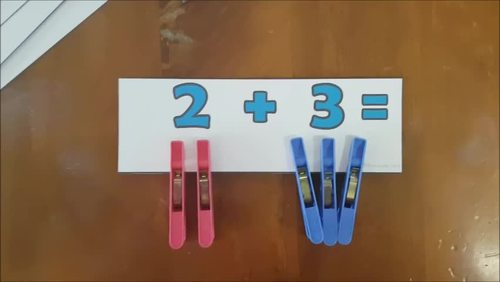# Peg Addition Facts to 10 - Commutative PropertiesSubject
Resource Type
File Type

Zip

(7 MB|40 pages)
Standards
• Product Description
• Standards

A hands on and visual way for students to practise and consolidate their understanding of the commutative properties of addition. Includes all addition facts within 10, including facts to 10 and plus zero facts.

Print double sided (long edge binding) addition fact cards and use pegs (not included) for a hands on activity so your students develop and consolidate their understanding of addition commutative property.

Use as a whole class demonstration, in Math Centres and in small groups.

Be sure to watch the video preview for a demo and a look inside!

What's Inside

→All addition facts up to 10, including 10 facts and add zero facts.

→All addition facts in colour and in black and grey.

RELATED PRODUCTS

Tic Tac Toe - An Addition Game (With a twist!)

Number Cross Out - An Addition Game

For more teaching ideas, freebies and resources, click on the

Like this resource? Please leave a review and earn TpT credit points you can use for future purchases.

This publication may only be printed by the original purchaser for use within their class(es). No part of this publication may be edited, shared, reproduced, distributed, re-sold or transmitted in any form or by any means, including photocopying, printing, recording, or other electronic or mechanical methods, without the prior written permission of the publisher. Violations are subject to the penalties of the digital Millennium Copyright Act.

Add and subtract within 20, demonstrating fluency for addition and subtraction within 10. Use strategies such as counting on; making ten (e.g., 8 + 6 = 8 + 2 + 4 = 10 + 4 = 14); decomposing a number leading to a ten (e.g., 13 - 4 = 13 - 3 - 1 = 10 - 1 = 9); using the relationship between addition and subtraction (e.g., knowing that 8 + 4 = 12, one knows 12 - 8 = 4); and creating equivalent but easier or known sums (e.g., adding 6 + 7 by creating the known equivalent 6 + 6 + 1 = 12 + 1 = 13).
Apply properties of operations as strategies to add and subtract. If 8 + 3 = 11 is known, then 3 + 8 = 11 is also known. (Commutative property of addition.) To add 2 + 6 + 4, the second two numbers can be added to make a ten, so 2 + 6 + 4 = 2 + 10 = 12. (Associative property of addition.)
Fluently add and subtract within 5.
For any number from 1 to 9, find the number that makes 10 when added to the given number, e.g., by using objects or drawings, and record the answer with a drawing or equation.
Represent addition and subtraction with objects, fingers, mental images, drawings, sounds (e.g., claps), acting out situations, verbal explanations, expressions, or equations.
Total Pages
40 pages
N/A
Teaching Duration
N/A
Report this Resource to TpT
Reported resources will be reviewed by our team. Report this resource to let us know if this resource violates TpT’s content guidelines.